## Quiz 11 : International Debt FinancingLooking for Finance Homework Help?# Quiz 11 : International Debt Financing

a. First, calculate the final payment in terms of Yen by multiplying the final principal payment in terms of US dollars i.e. USD115.956 million by the actual 5-year forward rate of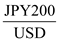as follows.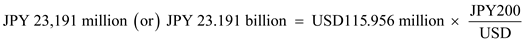The final payment (In JPY) is less than the original principal amount of JPY25 billion. Thus, we can say that it is not exactly a fair deal for the investor as the final payment is less than the face value of the bond. It is obvious that the stated coupon of 7.75% is more than the comparable fixed-rate Euroyen bond of 6.375%, hence actual final payment of JPY 25 billion should not be expected unless the bond's rate of return would be the same as coupon of 7.75%. If we choose to hedge the bond and find the internal rate of return of all the cash flows, we will get the value y as an IRR, which is the value when the discounted yen cash flows are equals to the cost of the bonds:The answer to the above equation can be found by using Excel IRR function. For IRR function in Excel, first click the function wizard, fx, then financial, IRR, and then OK. Enter the range of future cash flows in the IRR function as "Values". Click Ok, and the result is IRR of the project. The internal rate of return on the bond is 6.48% which is more than the comparable fixed-rate Euroyen bond of 6.375%, hence it can be a good deal for investors if they opt hedging with actual 5-year forward rate of. Internal rate of return: It is the discount rate at what the net present value of all cash flows from a particular project equal to zero. Generally, IRR will be used in capital budgeting. b. At the time of maturity i.e. in August 1990, if the exchange rate was JPY144/USD, to find a fair deal we will first find out the return that the investors will have in unhedged position. We can calculate the final payment in terms of Yen by multiplying the final principal payment in terms of US dollars i.e. USD115.956 million by the actual forward rate of JPY144/USD as follows.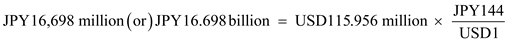The final payment (In JPY) is almost ¥7 billion less than the original principal amount of JPY25 billion at the forward rate of JPY144/USD in August 1985. This loss was due to the fact that yen appreciated by more than whatever expected by the forward rate. Thus, we can say that it is not exactly a fair deal for the investor as the final payment is less than the face value of the bond. We should find actual internal rate of return in unhedged position of all the cash flows, we will get the value y as an IRR, which is the value when the discounted yen cash flows are equals to the cost of the bonds: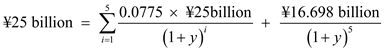The answer to the above equation can be found by using Excel IRR function. For IRR function in Excel, first click the function wizard, fx, then financial, IRR, and then OK. Enter the range of future cash flows in the IRR function as "Values". Click Ok, and the result is IRR of the project. The internal rate of return on the bond is 1,28% which is very much less than the comparable fixed-rate Euroyen bond of 6.375%, hence, it is not at all a good deal for investors.

There are three main sourcing of financing for any firm. These are as follows: 1. Internally generated Fund: These are the funds that are generated or kept within the firm like reserves and retained earnings. 2. Debt Financing: These are also considered as external sources of funds where the cash is generated externally from the debt markets. In debt financing, firm issues debt stock to the investors with the help of some financial intermediaries. Debt financing consist of debt securities traded locally foreign bond market, euro market and bank loans. 3. Equity Financing: Equity financing is also considered as the internal sources of financing where the cash is generated externally by issuing the equity stocks and selling it to the customers. These stocks are also traded in secondary markets.

a) Expected interest rate cost for loans in EUR and GBP : The expected interest rate cost includes both the interest costs and as well as the expected capital gains or losses on the various currency positions. The expected cost for the EUR will be: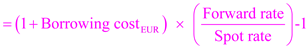Substitute the values in the formula: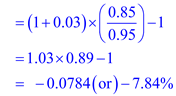The expected cost for the GBP will be calculated by the formula: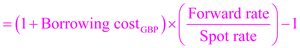Substitute the values in the formula: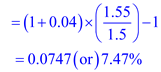The expected cost for the EUR and GBP will be -7.84% and 7.47% respectively. b) The exchange rates satisfying the uncovered Interest Rate Parity is calculated as follows: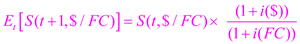Where, FC represents the foreign currency either the EUR or the GBP. For the euro, the exchange rate i.e. USD/EUR rate satisfying the uncovered Interest Rate Parity is calculated as follows: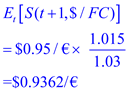For the pound, the exchange rate i.e. USD/GBP rate satisfying the uncovered Interest Rate Parity is calculated as follows: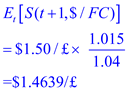The exchange rates satisfying the uncovered Interest Rate Parity for the Euro is \$0.9362/€ and for pound is \$1.4369/£. c) As per the calculations in part a, the interest cost is lowest in EUR. But if we see part b, the computed "break-even" exchange rates are closely related to the forward rates as per the market. Also, we cannot say so surely that whatever the lesser expected interest cost is will actually realized. So borrowing in low interest countries can result in savings. The company can do some investigation about in which country the credit spread is at its minimum and can borrow in that country and to stabilize the foreign exchange risk it can hedge back to dollars. If GBA generates its cash flows in UK and continental Europe, the borrowing in their currencies will lead to natural hedge.

There is no answer for this question